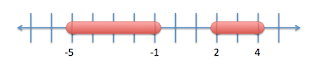## Sunday, February 21, 2016

One simple thing that comes up time and time again is the use of the greater than sign as part of a conditional while programming. Removing it cleans up code, here's why:

## Conditionals can be confusing

Let's say that I want to check that something is between 5 and 10.
There are many ways I can do this

All of these mean the same thing... Wait, did I actually do all that right? Sorry, one of those is incorrect. Go ahead and find out which one, I'll wait...

If you remove the use of the greater than sign then only 2 options remain:
(x < 10 && 5 < x)
which is a stupid option because it implies 10 < 5 and
(5 <&& x < 10)

This is a nice way of expressing "x is between 5 and 10" because it is literally between 5 and 10.

It's also a nice way of expressing that "x is outside the limits of 5 and 10"
(x < 5 || 10 < x)

Again, this expresses it nicely because x is literally outside of 5 to 10.

Simple. Clear. Consistent.

This is such a nice way to express numbers I wonder why programming languages allow for the greater than sign ( > ) at all.

But why is this so expressive?

## The Number line

Here's how you represent between 5 and 10 on a number line vs code:
(5 <&& x < 10)

Here's how you represent outside of  5 and 10 on a number line vs code:
(x < 5 || 10 < x)

On a number line everything to the left is less than the numbers to the right, so these two ways of representing the relationship between things matches up.

## Combinatorics

This problem gets much worse as the conditional grows. For example((-5 <&& x < -1) || (2 <&& x < 4))

Has 15 other possible ways to be expressed if you  include the greater than sign and don't make your expressions conform to the number line.